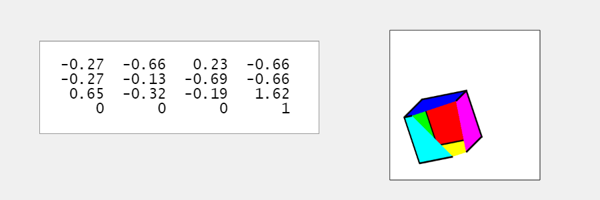# The Computer Graphics Matrix and a Cube

Use a cube instead of the Utah Teapot in my previous post. I was pleasantly suprised by the final screen shot.

### Contents

#### RGB cube

Three faces of our cube are colored with red, green and blue. The opposite faces have the complentary colors, cyan, magenta and yellow. Initially, only the yellow face is visible.

    Cube = grafix('6','rgb');   % Platonic solid with 6 faces.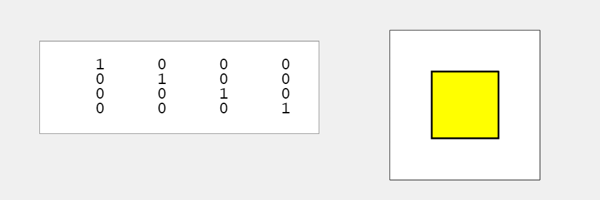#### Rz

    Rz = R_z(45);
apply(Cube,Rz)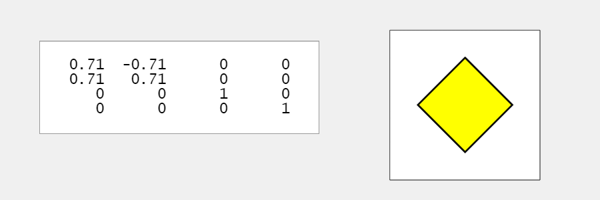#### Rx*Rz

    Rx = R_x(60);
M = Rx*Rz;
apply(Cube,M)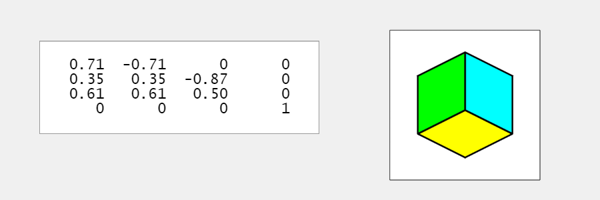#### Rz*Rx

    M = Rz*Rx;
apply(Cube,M)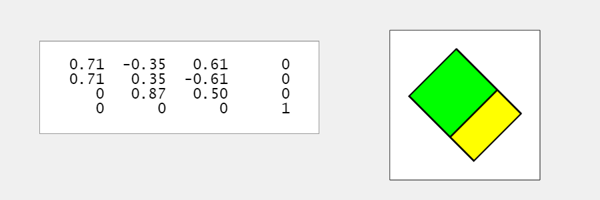#### Ry

    Ry = R_y(-120);
apply(Cube,Ry)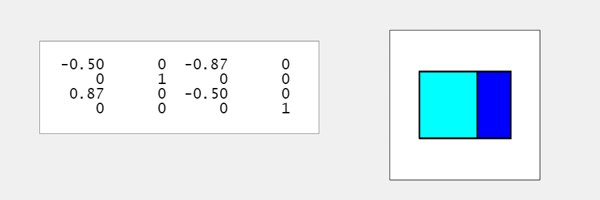#### Tx

    Tx = T_x(2.5);
apply(Cube,Tx)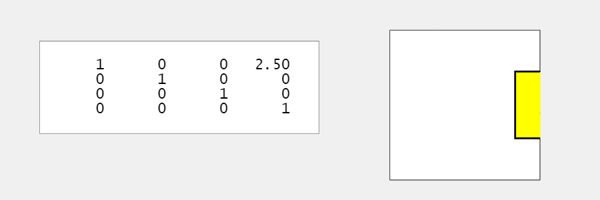#### Sxyz

    Sxyz = S_xyz(0.75);
apply(Cube,Sxyz)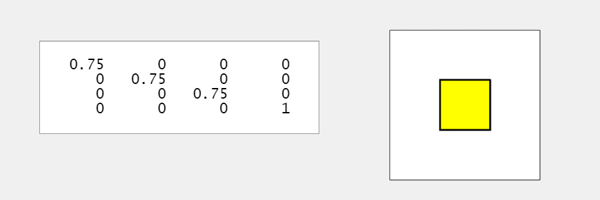#### All together

    M = Sxyz*Tx*Ry*Rz*Rx;
apply(Cube,M)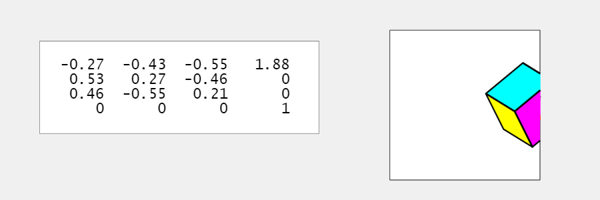M = Rx*Rz*Ry*Tx*Sxyz;
apply(Cube,M)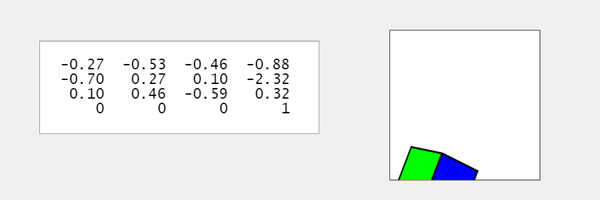#### Clipped

Finally, a scrambled order. The cube is clipped by the plot box and we see though the interior to the opposite faces. All six colors are visible.

    M = Rz*Ry*Sxyz*Tx*Rx;
apply(Cube,M)